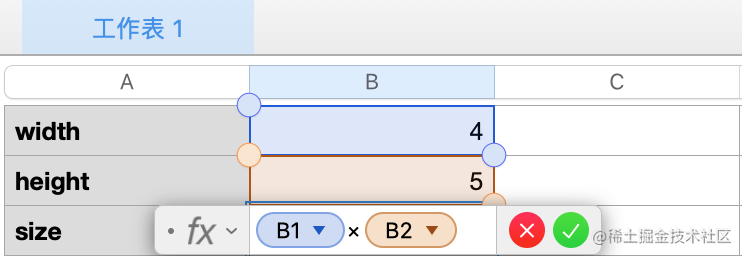# Vue3 响应式原理探索Part 1 - 20行代码实现响应式

“这是我参与更文挑战的第2天，活动详情查看： 更文挑战

## 响应式``````let width = 4
let height = 5
let size = width * height

// size
// 20

width = 5

// size
// 20

height = 6

// size
// 20

• 如何感知到值的变化
• 值变化时，该执行哪些函数，是求 size 还是求周长
• 值变化时，如何通知函数执行

## 手动触发

``````let param = { width: 4, height: 5 };
let size = 0;

let effect = () => {
size = param.width * param.height
}

track();
effect();

``````let dep = new Set() // 一个用来存储 effect 类似函数的列表

function track() {
dep.add(effect) // 存储当前的 effct
}

function trigger() {
dep.forEach(effect => effect()) // 执行所有的 effect
}

``````let param = { width: 4, height: 5 };
let size = 0;

let effect = () => {
size = param.width * param.height
}

let dep = new Set() // 一个用来存储 effect 类似函数的列表

function track() {
dep.add(effect) // 存储当前的 effct
}

function trigger() {
dep.forEach(effect => effect()) // 执行所有的 effect
}

track(); // 添加追踪

effect();
console.log(size); // => 20

param.width = 5;
trigger(); // 手动触发
console.log(size); // => 25

param.width = 6;
trigger(); // 手动触发
console.log(size); // => 30

bigo，一个傻瓜版本的响应式实现了。

## 思考或疑惑

• 每次值变化都需要手动 `trigger`, 太弱鸡了，为什么不能自动的执行
• 当应用更复杂时，譬如：
• param 有 N 个字段，有些字段需要响应，有些字段不需要响应，现在这样做是否太简单粗暴了。
• 有多个类似 param 的 object 需要响应时，又怎么办，一个 Set 是否太简单了